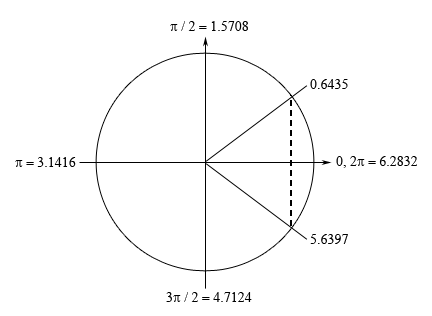Paul's Online Notes
Home / Calculus I / Review / Trig Equations with Calculators, Part I
Show Mobile Notice Show All Notes Hide All Notes
Mobile Notice
You appear to be on a device with a "narrow" screen width (i.e. you are probably on a mobile phone). Due to the nature of the mathematics on this site it is best views in landscape mode. If your device is not in landscape mode many of the equations will run off the side of your device (should be able to scroll to see them) and some of the menu items will be cut off due to the narrow screen width.

### Section 1.5 : Solving Trig Equations with Calculators, Part I

2. Find the solution(s) to $$\displaystyle 6 + 5\cos \left( {\frac{x}{3}} \right) = 10$$ that are in $$\left[ {0,38} \right]$$. Use at least 4 decimal places in your work.

Show All Steps Hide All Steps

Hint : Find all the solutions to the equation without regard to the given interval. The first step in this process is to isolate the cosine (with a coefficient of one) on one side of the equation.
Start Solution

Isolating the cosine (with a coefficient of one) on one side of the equation gives,

$\cos \left( {\frac{x}{3}} \right) = \frac{4}{5}$
Hint : Using a calculator and your knowledge of the unit circle to determine all the angles in the range $$\left[ {0,2\pi } \right]$$ for which cosine will have this value.
Show Step 2

First, using our calculator we can see that,

$\frac{x}{3} = {\cos ^{ - 1}}\left( {\frac{4}{5}} \right) = 0.6435$

Now we’re dealing with cosine in this problem and we know that the $$x$$-axis represents cosine on a unit circle and so we’re looking for angles that will have a $$x$$ coordinate of $$\frac{4}{5}$$. This means that we’ll have angles in the first (this is the one our calculator gave us) and fourth quadrant. Here is a unit circle for this situation.From the symmetry of the unit circle we can see that we can either use –0.6435 or $$2\pi - 0.6435 = 5.6397$$ for the second angle. Each will give the same set of solutions. However, because it is easy to lose track of minus signs we will use the positive angle for our second solution.

Hint : Using the two angles above write down all the angles for which cosine will have this value and use these to write down all the solutions to the equation.
Show Step 3

From the discussion in the notes for this section we know that once we have these two angles we can get all possible angles by simply adding “$$+ \,2\pi n$$ for $$n = 0, \pm 1, \pm 2, \ldots$$” onto each of these.

This then means that we must have,

$\frac{x}{3} = 0.6435 + 2\pi n\hspace{0.25in}{\mbox{OR }}\hspace{0.25in}\frac{x}{3} = 5.6397 + 2\pi n\hspace{0.25in}n = 0, \pm 1, \pm 2, \ldots$

Finally, to get all the solutions to the equation all we need to do is multiply both sides by 3 and we’ll convert everything to decimals to help with the final step.

\begin{align*}x & = 1.9305 + 6\pi n & \hspace{0.25in}{\mbox{OR }}\hspace{0.25in} x & = 16.9191 + 6\pi n\hspace{0.25in} & n = 0, \pm 1, \pm 2, \ldots \\ & = 1.9305 + 18.8496n & \hspace{0.25in}{\mbox{OR }}\hspace{0.35in} & = 16.9191 + 18.8496n\hspace{0.25in} & n = 0, \pm 1, \pm 2, \ldots \end{align*}
Hint : Now all we need to do is plug in values of $$n$$ to determine which solutions will actually fall in the given interval.
Show Step 4

Now let’s find all the solutions. First notice that, in this case, if we plug in negative values of $$n$$ we will get negative solutions and these will not be in the interval and so there is no reason to even try these. So, let’s start at $$n = 0$$ and see what we get.

$\begin{array}{lclcl}{n = 0:} & \hspace{0.25in} & {x = 1.9305\,}& \hspace{0.25in} {{\mbox{OR}}} \hspace{0.25in} &{x = 16.9191}\\{n = 1:} & \hspace{0.25in} & {x = 20.7801\,}& \hspace{0.25in} {{\mbox{OR}}} \hspace{0.25in} &{x = 35.7687}\end{array}$

Notice that with each increase in $$n$$ we were really just adding another 18.8496 onto the previous results and by doing this to the results from the $$n = 1$$ step we will get solutions that are outside of the interval and so there is no reason to even plug in $$n = 2$$.

So, it looks like we have the four solutions to this equation in the given interval.

$\require{bbox} \bbox[2pt,border:1px solid black]{{x = 1.9305,\,\,16.9191,\,\,20.7801,\,\,35.7687}}$

Note that depending upon the amount of decimals you used here your answers may vary slightly from these due to round off error. Any differences should be slight and only appear around the 4th decimal place or so however.